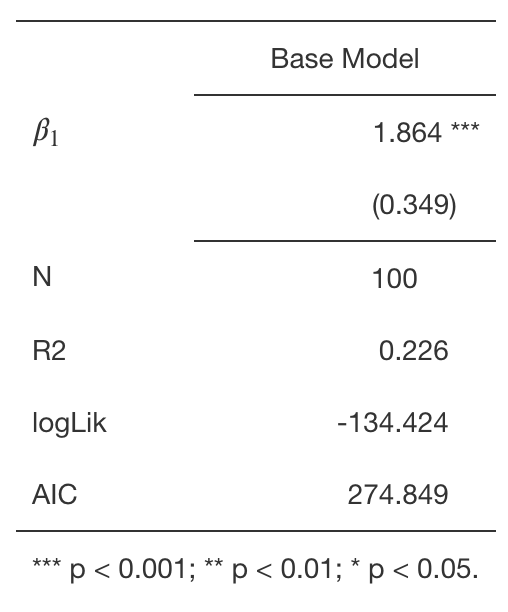# displaying math and greek symbols with the huxtable r library

I love using David Hugh-Jones' huxtable library in R. But I hate forgetting how to include math symbols in the resulting tables. Here's how to do it.

Huxtable is an extensive R package that prints tables in R. Its creator, David Hugh-Jones, documents the package extremely well and responds to questions and concerns about the package on Github and StackOverflow immediately. He continually incorporates feature requests and, even in my relatively short time using R, he has made the already powerful library even more so.

The huxreg function included in the library provides an indispensable tool in my reproducible workflow. The function gives users a powerful means to output the results of regression equations. These can easily be output into a number of different formats, most notably HTML and LaTeX with, appropriately enough, the to_html() and to_latex() functions.

The huxreg function works by taking model objects that have tidy methods defined (and can be a named list to name the models) and outputs the results. The coefs parameter allows the user to select, order, and rename the parameters. Take the example below of a simple regression model. I want to display only the coefficient representing the influence of variable x and I want to call it beta_1. I could use the following:

library(huxtable)

x <- runif(100)
y <- rnorm(100, 1 + 2 * x)
m <- lm(y ~ x)

huxreg(list("Base Model" = m), coefs = c("beta_1" = "x"))


And the output would be:Example of huxtable table in with Greek symbol

I often find that I want to use the actual Greek letters or other math symbols in model or variable names. Instinctively, I always try to use the general Markdown syntax by enclosing math in dollar signs $$$\ldots$$$, e.g., $\beta_1$.

When I use that syntax in Rmarkdown files, however, it displays the enclosing dollar signs and LaTeX code rather than the actual math that I desire.

I can't remember where I found the solution, but I somewhere Hughes-Jones suggested using the alternate math delimiters $$\(\ldots$$\) rather than $$$\ldots$$$. Using that delimiter displays the format correctly in the resulting Markdown. But, here, there's some tricky syntax because R (like lots of languages) uses the backslash character, \, as an escape character. As a result, to get the symbol to display correctly, we need to use double backslashes anywhere we want a backslash. As a result, we end up with the following code and $$\beta_1$$ listed as the variable in the table:

huxreg(list("Base Model" = m), coefs = c("\$$\\beta_1\$$" = "x"))


A final problem comes if we want the variable name to be $$\beta_1$$ in a LaTeX table that we might wish to save as a file. huxreg, by default, escapes the characters so that what you type is what you see in the file. In the case of LaTeX, however, we do not want the actual text "\$$\\beta_1\$$", we want LaTeX to read the $$ and $$ as math delimiters not as text. Therefore, we need to tell huxtable to read the contents as LaTex and not to escape the contents of the cell. To do that, we se the escape_contents parameter on the cell to FALSE using the set_escape_contents() function and defining the cell (row 2, col 1).

hux <- huxreg(list("Base Model" = m), coefs = c("\$$\\beta_1\$$" = "x")) |>
set_escape_contents(2, 1, FALSE) |>
quick_pdf(file = "huxtable-output.pdf")


To demonstrate that it works, I rendered a PDF using the quick_pdf() function. In reproducible research, however, it may be better to export the table to LaTeX or HTML to include in a manuscript later.

I hope that helps some of you learn about the huxtable library and helps me to remember to use the $$\(\ldots$$\) delimiters to display math symbols in Rmarkdown.

#### Pingbacks

Pingbacks are closed.